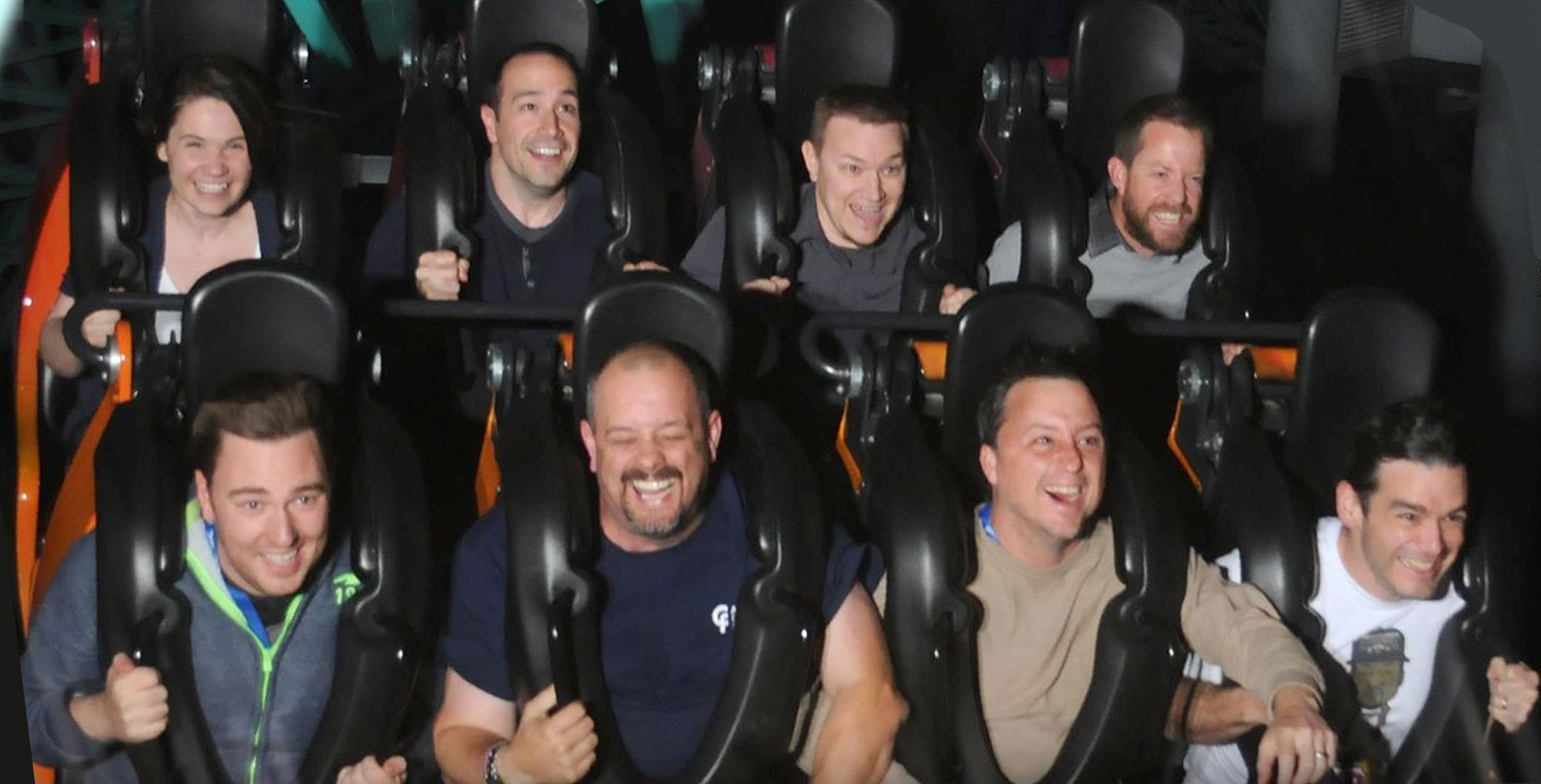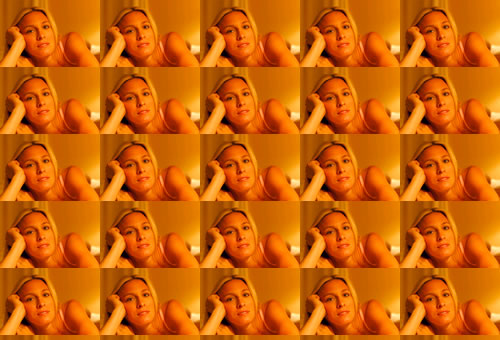Ben Nadel at cf.Objective() 2014 (Bloomington, MN) with: Emily Christiansen ( @egchristiansen ) , Simon Free ( @simonfree ) , Dave Ferguson ( @dfgrumpy ) , Joel Hill ( @Jiggidyuo ) , Dan Wilson ( @DanWilson ) , Jeff McDowell ( @jeff_s_mcdowell ) , and Brian Rinaldi ( @remotesynth )

# Creating A Tiled Image Background With TileImage()

By on
Tags:

I've added a new function, TileImage(), to Ray Camden's and my ImageUtils.cfc. This new function will completely tile the given graphic onto the given ColdFusion image canvas. Think of this as a repeated background image on a web site or a background pattern on a FireWorks shape. The method looks like this:

TileImage( Image, TileImage [, X [, Y ]] )

#### Image

The image onto which we are tiling the other image.

#### TileImage

The image that we are going to be tiling onto our canvas

#### X

The optional X coordinate from which we are going to start tiling. This defaults to zero but can be set to positive or negative number.

#### Y

The optional Y coordinate from which we are going to start tiling. This defaults to zero but can be set to positive or negative number.

Let's take a quick look at this baby in action:

``````<!--- Create imageUtils.cfc instance. --->
<cfset objUtils = CreateObject( "component", "imageUtils" ).Init() />

<!--- Create a blank canvas. --->
<cfset objImage = ImageNew( "", 500, 340, "rgb" ) />

<!--- Read in the image that we are going to tile. --->
<cfimage
source="./subject.jpg"
name="objTile"
/>

<!--- Scale the tile image so that it will tile better. --->
<cfimage
action="resize"
source="#objTile#"
height="20%"
width="20%"
name="objTile"
/>

<!---
Tile the image onto our canvas. You can supply an optional
starting X and Y coordniate for the pasting operations; I
have included them in this method call and just set them
to zero (same as if I didn't include them at all).
--->
<cfset objUtils.TileImage(
objImage,
objTile,
0,
0
) />

<!--- Write to broser. --->
<cfimage
action="writetobrowser"
source="#objImage#"
/>
``````

Here, we are creating a blank canvas and then tiling our `subject.jpg` image onto it. We are scaling down the tile image just so that it tiles, otherwise it would be roughly the same size as our blank canvas. Running the above code, we get the following output:Here is the code for this ColdFusion user define function for image manipulation:

``````<cffunction
name="TileImage"
access="public"
returntype="any"
output="false"
hint="Takes your ColdFusion image object and tiles the given image over it.">

<!--- Define arguments. --->
<cfargument
name="Image"
type="any"
required="true"
hint="The ColdFusion image onto which we are going to tile our graphic."
/>

<cfargument
name="TileImage"
type="any"
required="true"
hint="The image that we are going to tile onto our ColdFusion image."
/>

<cfargument
name="X"
type="numeric"
required="false"
default="0"
hint="The X point at which we are going to start our tiling."
/>

<cfargument
name="Y"
type="numeric"
required="false"
default="0"
hint="The Y point at which we are going to start our tiling."
/>

<!--- Define the local scope. --->
<cfset var LOCAL = {} />

<!---
Check to see what kind of value the tile image is.
If it is not an image, then we have to create an
image with it such that we can more easily get the
height and width dimensions.
--->
<cfif NOT IsImage( ARGUMENTS.TileImage )>

<!--- Read in the image file. --->
<cfimage
source="#ARGUMENTS.TileImage#"
name="ARGUMENTS.TileImage"
/>

</cfif>

<!--- Get the image dimensions. --->
<cfset LOCAL.ImageDimensions = {
Width = ImageGetWidth( ARGUMENTS.Image ),
Height = ImageGetHeight( ARGUMENTS.Image )
} />

<!--- Get the tile image dimensions. --->
<cfset LOCAL.TileDimensions = {
Width = ImageGetWidth( ARGUMENTS.TileImage ),
Height = ImageGetHeight( ARGUMENTS.TileImage )
} />

<!---
Now that we have our dimensions, we need to figure
out where we need to start tiling. We need to make
sure that we start at or before ZERO in both of
the axis.
--->
<cfset LOCAL.StartCoord = {
X = ARGUMENTS.X,
Y = ARGUMENTS.Y
} />

<!--- Find the starting X coord. --->
<cfloop condition="(LOCAL.StartCoord.X GT 0)">

<!--- Subtract the width of the tiled image. --->
<cfset LOCAL.StartCoord.X -= LOCAL.TileDimensions.Width />

</cfloop>

<!--- Find the starting Y coord. --->
<cfloop condition="(LOCAL.StartCoord.Y GT 0)">

<!--- Subtract the width of the tiled image. --->
<cfset LOCAL.StartCoord.Y -= LOCAL.TileDimensions.Height />

</cfloop>

<!---
ASSERT: At this point, our StartCoord has the {X,Y}
point at which we need to begin our tiling.
--->

<!---
Set the current coordindate of pasting to be the
start point that we calcualted above. We will be
updating this value as we tile the image.
--->
<cfset LOCAL.PasteCoord = StructCopy( LOCAL.StartCoord ) />

<!---
Now, we want to keep looping until the Y coord
of our pasting is greater than the heigh of our
target ColdFusion image.
--->
<cfloop
index="LOCAL.PasteCoord.Y"
from="#LOCAL.StartCoord.Y#"
to="#LOCAL.ImageDimensions.Height#"
step="#LOCAL.TileDimensions.Height#">

<!---
As we loop over the Y coordinate, we want to
also keep looping until our X pasting coordinate
goes beyond the width of the target ColdFusion
image.
--->
<cfloop
index="LOCAL.PasteCoord.X"
from="#LOCAL.StartCoord.X#"
to="#LOCAL.ImageDimensions.Width#"
step="#LOCAL.TileDimensions.Width#">

<!---
Paste the tile image onto the target
ColdFusion image at the given X,Y
coordinate.
--->
<cfset ImagePaste(
ARGUMENTS.Image,
ARGUMENTS.TileImage,
LOCAL.PasteCoord.X,
LOCAL.PasteCoord.Y
) />

</cfloop>

</cfloop>

<!--- Return the updated image. --->
<cfreturn ARGUMENTS.Image />
</cffunction>
``````

Want to use code from this post? Check out the license.